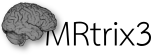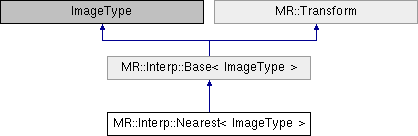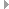Developer documentation Version 3.0.3-105-gd3941f44
MR::Interp::Nearest< ImageType > Class Template Reference

This class provides access to the voxel intensities of an Image, using nearest-neighbour interpolation. More...

`#include "interp/nearest.h"`

Inheritance diagram for MR::Interp::Nearest< ImageType >:Public Member Functions inherited from MR::Transform
void * operator new (std::size_t size)

void * operator new[] (std::size_t size)

void operator delete (void *ptr)

void operator delete[] (void *ptr)

An object for transforming between voxel, scanner and image coordinate spaces. More...

Transform (const Transform &)=default

Transform (Transform &&)=default

Transformoperator= (const Transform &)=delete

Transformoperator= (Transform &&)=deleteStatic Public Member Functions inherited from MR::TransformPublic Attributes inherited from MR::Transform
const Eigen::DiagonalMatrix< default_type, 3 > voxelsize

const transform_type voxel2scanner

const transform_type scanner2voxel

const transform_type image2scanner

const transform_type scanner2imageProtected Member Functions inherited from MR::Interp::Base< ImageType >
template<class VectorType >
bool set_out_of_bounds (const VectorType &pos)

void set_out_of_bounds (const bool value)

template<class VectorType >
Eigen::Vector3d intravoxel_offset (const VectorType &pos)Protected Attributes inherited from MR::Interp::Base< ImageType >
default_type bounds 

bool out_of_bounds

## Detailed Description

template<class ImageType>
class MR::Interp::Nearest< ImageType >

This class provides access to the voxel intensities of an Image, using nearest-neighbour interpolation.

Interpolation is only performed along the first 3 (spatial) axes. The (integer) position along the remaining axes should be set using the template Image class. The spatial coordinates can be set using the functions voxel(), image(), and scanner(). For example:

auto input = Image<float>::create (Argument);
// create an Interp::Nearest object using input as the parent data set:
Interp::Nearest<decltype(input) > interp (input);
// set the scanner-space position to [ 10.2 3.59 54.1 ]:
interp.scanner (10.2, 3.59, 54.1);
// get the value at this position:
float value = interp.value();
Definition: image.h:192

The template input class must be usable with this type of syntax:

int xsize = input.size(0); // return the dimension
int ysize = input.size(1); // along the x, y & z dimensions
int zsize = input.size(2);
float v[] = { input.spacing(0), input.spacing(1), input.spacing(2) }; // return voxel dimensions
input.index(0) = 0; // these lines are used to
input.index(1)--; // set the current position
input.index(2)++; // within the data set
float f = input.value();
transform_type M = input.transform(); // a valid 4x4 transformation matrix
Eigen::Transform< default_type, 3, Eigen::AffineCompact > transform_type
the type for the affine transform of an image:
Definition: types.h:234

Definition at line 68 of file nearest.h.

The documentation for this class was generated from the following file: